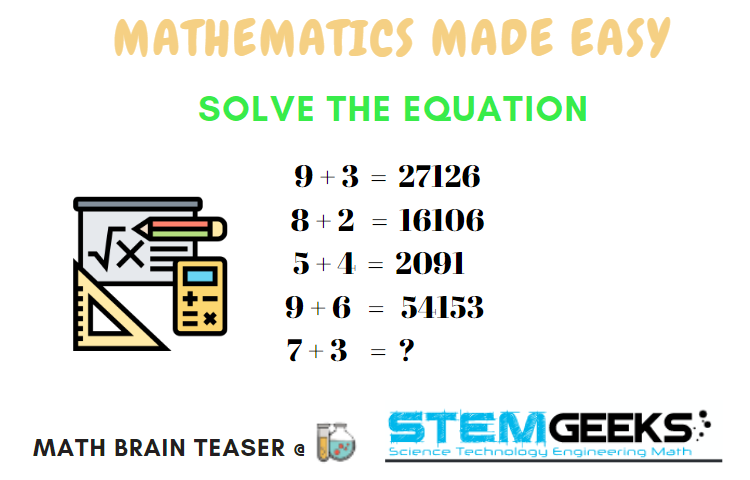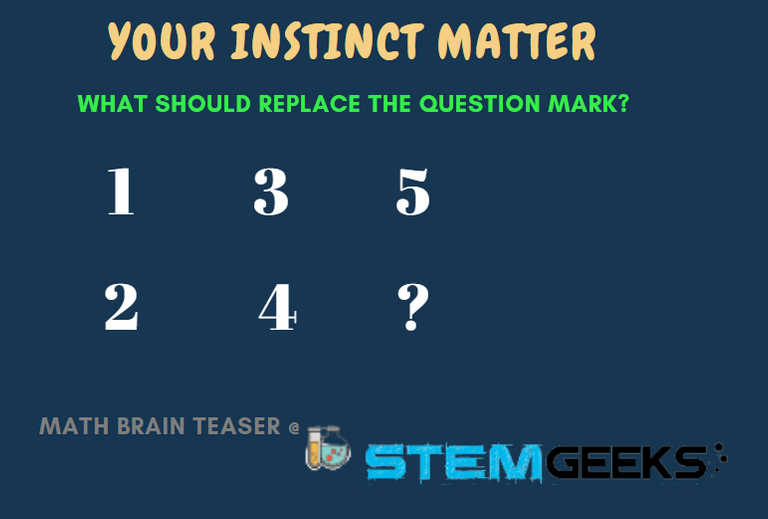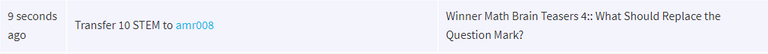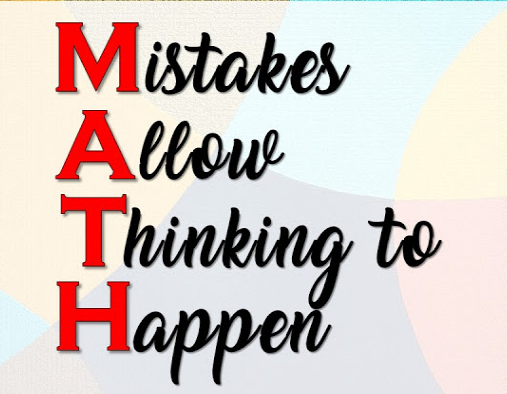# Math Brain Teasers 5:: Solve the Equation; What Do You Get?

in STEMGeeks2 years ago (edited)

Hey All;

It's time for the FIFTH:: Math Brain Teaser & this time we have a simple equation to solve. Let me assure you that it's not that complicated and you will not require any calculator to solve this equation; it's that SIMPLE.

Have a look at the following FOUR "Equations" below. And try to the answer to the 5th Equation?What you do require is to carefully look at the equation and try finding the logic to arrive at the answer.

Hint:: I've already given a hint; we have a simple logic to solve this equation. And I promise you dont require any calculator for it.. Its simple Addition; Multiplication; Subtraction is all that is required to solve this equation.

### Math Brain Teasers 4:: What Should Replace the Question Mark? - Solved with Explanation

Let's have a look at the answers and the math/logic involved in finding:: What Should Replace the Question Mark? for our FOURTH Math Brain Teasers 4 Question.### Logic to Finding; what should Replace the Question Mark?

Actually; the truth be told there was no logic to be applied for this Math Brain Teaser. We only had to think of a situation where these numbers are to be seen on a daily basis. Did you guess it NOW?

If not then, let me Reval the Answer. This puzzle was tied to the CAR liver and if you carefully observe the Car lever as shown in the image;The question mark was to be Replaced with the letter - "R" & not with the Number - 6

### STEM token GiveAway

I'll be again doing a giveaway of STEM tokens to the lucky random winner with the correct answer. We had 7 entries and out of which there was only one entry that made it to the right answer. Hence the Winner for the Math Brain Teasers 4 is @amr008Congratulations @amr008;You WIN yourself 10 STEM tokens; worth 2.3+ HIVE. You should be having the rewards in your STEM Wallet Soon.

### Math Quote for the Day::

Here is the motivation to solve this Math Brain Teasers 5:: Solve the Equation; What Do You Get?Source::

If you like my work, then please spread the Word.. that we do have Math Brain Teasers competion here @ StemGeeks.

Best Regards

Posted with STEMGeeks

Sort:

Thanks @gungunkrishu .

Today's answer has already been given by @dobro2020 but still I will try to explain how the answer is arrived at-

7*3 then 7+3 and then 7-3 .

Good explanation... but I think still disclosing the answer in comment is not fair... let ithers also test their brain....
!ENGAGE 25

Ah you are right .

Thank you for your engagement on this post, you have recieved `ENGAGE` tokens.

21104 i think so jajaja

Posted using Dapplr

Ah well you beat me to it. It is right hehe.

Very fast...
!ENGAGE 20

Thank you for your engagement on this post, you have recieved `ENGAGE` tokens.

21104

I got it after seeing 5+4=2091

This one is easy. The answer is 21104

Product, sum, and answer from subtraction in the answer digits. `7 + 3` = 21104